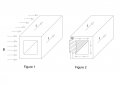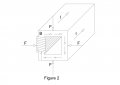# Force acting on a current carrying hollow conductor in a magnetic field

#### sss2009

Joined Sep 24, 2019
2
It is known that when a current carrying conductor is placed within a magnetic field and current flows perpendicular to the magnetic field, a force is exerted on the conductor.

In figure 1, a square hollow conductor is shown in which DC current, I, is flowing perpendicular to the magnetic field, B. By Flemings left hand rule, the force acts downwards on the whole conductor.

What happens when the same current carrying conductor is subject to a magnetic field generated within? In figure 2, the same hollow square conductor is wrapped with an energized coil and magnetic field is generated within its cross section. DC Current is made to flow through the conductor in the same way as figure 1. Would a force be exerted perpendicular to each of the four sides of the square cross-section?#### Glenn Holland

Joined Dec 26, 2014
703
Faraday's Law says the force is always at a right angle to the direction of the current flow.

This applies to all shapes of the conductor. For the coil wrapped around an an iron core, the flux forms a loop so there is a field within the coil and also on the outside.So there is no net force on the wire. The field wraps around a straight wire and there is no net force.

#### sss2009

Joined Sep 24, 2019
2
Faraday's Law says the force is always at a right angle to the direction of the current flow.

This applies to all shapes of the conductor. For the coil wrapped around an an iron core, the flux forms a loop so there is a field within the coil and also on the outside.So there is no net force on the wire. The field wraps around a straight wire and there is no net force.
Dear Glenn,

The force acting on the conductor in Figure 1 is given by F = B I L, where L is the length of the conductor. This is my understanding from standard textbooks on the subject.What I really wanted to know was whether the same equation applies to each side of the square cross section in figure 2 (please see the revised figure attached)? Is that why there is no net force, since they cancel out each other?

#### Glenn Holland

Joined Dec 26, 2014
703
Dear Glenn,

The force acting on the conductor in Figure 1 is given by F = B I L, where L is the length of the conductor. This is my understanding from standard textbooks on the subject.View attachment 186837

What I really wanted to know was whether the same equation applies to each side of the square cross section in figure 2 (please see the revised figure attached)? Is that why there is no net force, since they cancel out each other?
Here's a good explanation of the interaction of current within any conductor and the resulting magnetic field commonly know as the Hall Effect:

https://en.wikipedia.org/wiki/Hall_effect

For a conductor placed in an external magnetic field, the current is diverted over to one side of the conductor. Or when current is flowing through a conductor, the resulting field is forced to the outside of the conductor. In that case the magnetic field resembles a tube surrounding the conductor.

The plasma in a lighting bolt is a particularly interesting example of the Hall Effect where the magnetic field surrounding the plasma produces a "Pinch Effect" that compresses the plasma into a "thread" of very high current density..

#### Duane Peter Wetick

Joined Mar 30, 2018
3
From Faraday, the magnetic force is at right angles to the flow of current, Right Hand Rule. Looking at the coil yoke construction on older TV sets CRT's (Cathode Ray Tubes), one can see the coils are shaped to provide an enclosed magnetic field envelope for converging or diverging the CRT's electron beam.

Cheers, DPW [Everything has limitations, and I hate limitations.]

#### MrAl

Joined Jun 17, 2014
10,891
Hello,

In the first drawing the force is obvious because it's a common situation.
In the second drawing it's a little less obvious but just look for the direction of B and the direction of I and you can see what happens. The force is restricted to the construction but there is still a force, it just may or may not move anything depending on how tight the coil is wrapped.
If the coil is very tight such as having it enclosed in epoxy, then the force is restricted so much that the coil wires barely move at all. But if the coil is even slightly loose not rapped really tight or epoxied then the coil itself will move slightly. Using an AC current, the coil will vibrate up and down slightly and even make an audible noise if the frequency is within the human hearing range.
Can the whole construction move? If the coil vibrates then the whole construction will vibrate and if placed on a very low friction surface with a slight incline then the whole construction will work it's way downhill. It will follow the incline though through gravity not by any magnetic force rule.
If you attach small semi flexible strips to the bottom and slant them on a 45 degree angle and place that on a low friction level surface, the up and down vibration movement combined with the ratcheting action of the strips will make it move in the same direction as the slants of the strips. Again however it will move in a direction based on the slant of the strips not on any magnetic force rule.

So you can get movement, just not for the same reason.